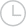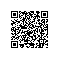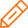# C语言：采用递归调用函数方法计算Fibonacci数列的前20项-问答-阿里云开发者社区-阿里云

## C语言：采用递归调用函数方法计算Fibonacci数列的前20项知与谁同2018-07-18 15:08:497206
C语言：采用递归调用函数方法计算Fibonacci数列的前20项取消 提交回答

• 我是管理员
2019-07-17 22:55:12
#include <stdio.h>
#define N 20
main(){
int f(int n);
int i;
for(i=1;i<=N;i++){
printf("%-6d",f(i));
if(i%5==0)
printf("\n");
}
printf("\n");
}

int f(int n){
if(n==1 || n==2)
return 1;
else
return (f(n-2)+f(n-1));
}
0 0
• 知与谁同
2019-07-17 22:55:12
long fibonacci(int n)
{ long t;
if (n=2)
{ t=1;
return t;
}
if (n=1)
{ t=1;
return t;
}
t=fibonacci(n-1)+fibonacci(n-2);
return t;

}

1、没有调试；
2、主 函数 自己 写；
0 0
• boxti
2019-07-17 22:55:12
#include <stdio.h>
#define N 20
main(){
int f(int n);
int i;
for(i=1;i<=N;i++){
printf("%-6d",f(i));
if(i%5==0)
printf("\n");
}
printf("\n");
}

int f(int n){
if(n==1 || n==2)
return 1;
else
return (f(n-2)+f(n-1));
}
0 0
• 行者武松
2019-07-17 22:55:12

使用递归进行操作时，关键是设置好递归条件。斐波那契数列的定义如下：f2 = f0 + f1 = 0 + 1 = 1，所以条件可以设置成当n==1或者n==2时，返回1。其它项则依次递归调用即可。 #include <stdio.h>
int f(int n){
if(n==1 || n==2)
return 1;
else
return (f(n-2)+f(n-1));
}
int main(){
const int num = 20;
int i;
for(i=1;i<=num;i++){
printf("%-6d",f(i));
if(i%5==0)
printf("\n");
}
printf("\n");
return 0;
}0 0添加回答使用钉钉扫一扫加入圈子
+ 订阅